# kalman¶

class nfoursid.kalman.Kalman(state_space: nfoursid.state_space.StateSpace, noise_covariance: numpy.ndarray)

Bases: object

Implementation  of a Kalman filter for a state-space model state_space: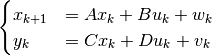The matrices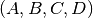are taken from the state-space model state_space. The measurement-noise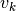and process-noise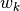have a covariance matrix noise_covariance defined as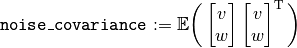Verhaegen, Michel, and Vincent Verdult. Filtering and system identification: a least squares approach. Cambridge university press, 2007.

actual_label = 'actual'

Label given to a column in self.to_dataframe, indicating measured values.

extrapolate(timesteps) pandas.core.frame.DataFrame

Make a timesteps number of steps ahead prediction about the output of the state-space model self.state_space given no further inputs. The result is a pd.DataFrame where the columns are self.state_space.y_column_names: the output columns of the state-space model self.state_space.

filtered_label = 'filtered'

Label given to a column in self.to_dataframe, indicating the filtered state of the Kalman filter.

next_predicted_corrected_label = 'next predicted (input corrected)'

Label given to a column in self.to_dataframe, indicating the predicted state of the Kalman filter corrected by previous inputs. The inputs to the state-space model are known, but not at the time that the prediction was made. In order to make a fair comparison for prediction performance, the direct effect of the input on the output by the matrix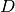is removed in this column.

The latest prediction will have np.nan in this column, since the input is not yet known.

next_predicted_label = 'next predicted (no input)'

Label given to a column in self.to_dataframe, indicating the predicted state of the Kalman filter under the absence of further inputs.

output_label = 'output'

Label given to an output column in self.to_dataframe.

plot_filtered(fig: matplotlib.figure.Figure)

The top graph plots the filtered output states of the Kalman filter and compares with the measured values. The error bars correspond to the expected standard deviations. The bottom graph zooms in on the errors between the filtered states and the measured values, compared with the expected standard deviations.

plot_predicted(fig: matplotlib.figure.Figure, steps_to_extrapolate: int = 1)

The top graph plots the predicted output states of the Kalman filter and compares with the measured values. The error bars correspond to the expected standard deviations.

The stars on the top right represent the steps_to_extrapolate-steps ahead extrapolation under no further inputs. The bottom graph zooms in on the errors between the predicted states and the measured values, compared with the expected standard deviations.

standard_deviation_label = 'standard deviation'

Label given to a standard deviation column in self.to_dataframe.

step(y: Optional[numpy.ndarray], u: numpy.ndarray)

Given an observed input u and output y, update the filtered and predicted states of the Kalman filter. Follows the implementation of the conventional Kalman filter in  on page 140.

The output y can be missing by setting y=None. In that case, the Kalman filter will obtain the next internal state by stepping the state space model.

 Verhaegen, Michel, and Vincent Verdult. Filtering and system identification: a least squares approach. Cambridge university press, 2007.

to_dataframe() pandas.core.frame.DataFrame

Returns the output of the Kalman filter as a pd.DataFrame. The returned value contains information about filtered and predicted states of the Kalman filter at different timesteps. The expected standard deviation of the output is given, assuming independence (!) of the state estimation error and measurement noise.

The rows of the returned dataframe correspond to timesteps. The columns of the returned dataframe are a 3-dimensional multi-index with the following levels:

1. The output name, in the list self.state_space.y_column_names.

2. An indication of whether the value is
• a value that was actually measured, these values were given to self.step as the y parameter,

• a filtered state,

• a predicted state given no further input or

• a predicted state where the effect of the next input has been corrected for. This column is useful for comparing prediction performance.

3. Whether the column is a value or the corresponding expected standard deviation.